Russian Journal of Earth Sciences
Vol. 3, No. 4, October 2001

# A mathematical model of the crustal waveguide

A. V. Karakin

VNIIGeosystem, Moscow

### Abstract

The two-layer model of the upper crust waveguide developed in [Karakin, 1990a; Karakin and Kambarova, 1997] is studied. The upper layer is poroelastic and the lower layer (the waveguide proper) can be in poroviscous or elastic dilatancy states. The model is based on the concept of two concurrent processes that are alternately active in the lower layer (waveguide): dilatancy and compaction. Horizontal tectonic forces displace the upper layer relative to the lower one. As a result, the porous space in the lower layer experiences dilatancy expansion, and fluids are sucked into the waveguide from upper and lower layers. The porous structure of the waveguide is then destroyed, which is accompanied by the transition of the system into the poroviscous state. The lithostatic pressure expels the fluids upward from the waveguide. This paper is devoted to the mathematical analysis of this model. A new formulation of the boundary value problem is proposed and a wave solution of the pertinent equations is given. Self excited waves in crustal waveguides are assumed to provide the driving mechanism of the vertical fluid migration in the upper crust. This migration gives rise to oil and gas deposits if the fluid flows strike impermeable anticlinal beds (traps). This model is a constituent part of the general concept of the mechanism responsible for mud volcanism. Faults cutting the traps and reaching the surface initiate the formation of mud volcanoes. Analysis of the waveguide model can provide constraints on the feeding conditions of mud volcanoes. The concept of the upper crust fluid regime proposed in the paper reconciles the hypotheses of the organic and inorganic origins of hydrocarbon accumulations. Hydrocarbon fluxes enter the crustal waveguide both from above (organic origin) and from below (inorganic origin). These fluid fluxes are reworked in the waveguide, after which they move upward. As a result, accumulated hydrocarbons display features of different origins.

### Introduction

Numerous studies [Fyfe et al., 1978; Grigoryev, 1971; Smith, 1968] provide evidence that the underground water forms a unified underground hydrosphere. Water (bound and free) in the crust is comparable in volume with ocean water and amounts to 4% of the crust volume. Such an amount of crustal water has a significant effect on all geological processes in the crust. One may naturally expect that the fluid effects are strongest in fractured, higher permeability layers, including waveguides. Therefore, the relevance of this problem is doubtless.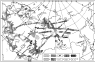Figure 1
Seismic, magnetotelluric and other studies show that the deep crust has a complex layered structure. Drilling data [Overdeep Kola Drillhole, 1984] and interpretation of deep seismic soundings [Seismic Models..., 1980] indicate that the crust is strongly fractured and saturated with fluids. The Kola hole yields evidence of the most fractured section in a depth interval from 7 to 10 km. Fracturing involves the entire crust and reaches the upper mantle. On the whole, the crust is composed of alternating rigid, seismically transparent and seismically opaque layers, the latter being waveguides. Data on the continental crust waveguides were summarized by Krasnopevtseva . Figure 1 presents a map showing crustal waveguides in the territory of the former USSR. According to seismic data, a waveguide is characterized by stronger attenuation and lower velocities of seismic waves. Magnetic measurements show that its electrical conductance ranges from 200 to 2000 S, which is much higher than the conductance of crustal layers above and below the waveguide. Although waveguides are widespread throughout the crust and occur at various depths, some regular features are recognized in their occurrence in the continental crust. They are more frequent at depths of 10-15 and 20-25 km. Their thickness varies from 1-2 to 15-17 km, most often amounts to 4-10 km and is typically higher in tectonically active zones. The velocity difference between the waveguide and surrounding rock masses varies from 0.1 to 1.0 km/s in the middle and upper crust. This velocity jump tends to increase with depth.

Gutenberg  supposed that seismic waveguides exist at asthenospheric depths. Afterward they were discovered in the upper mantle, lower crust and near the surface in rift zones. The low velocity zones were assumed to be related with the transition of the matter into amorphous state [Magnitsky, 1968] and with partial melting [Turcotte and Schubert, 1982]. This hypothesis suitable for the asthenosphere and consistent with its rheological properties was extended to the crust. However, temperatures in the crust (particularly in its upper part) are too low to account for the presence of melt inclusions throughout a large volume. Therefore, other hypotheses were also examined and majority of them related the waveguide properties to lithological distinctions of rocks (e.g. the presence of graphite-bearing rocks). The hypothesis on the lithological origin of waveguides encounters serious difficulties. One of the difficulties is the fact that the crust material is incessantly involved in upward and downward movements giving rise to folds and lithological unconformities, whereas waveguides occur, as a rule, horizontally at a fixed depth. This indicates that they are likely to be associated with the state, rather than composition, of the matter.

Comparison of seismic [Krasnopevtseva, 1978; Seismic Models..., 1980] and geoelectric [Vanyan, 1984; Vanyan and Shilovskii, 1983] data shows that low velocity and higher electrical conductivity zones often (but not always) coincide. The experimental data accumulated since the 1960s suggest that at depths not greater than 30 km these zones are most probably due to the presence in them of electrically conducting fluids [Vanyan, 1984]. The volume concentration of fluids in higher conductivity layers attains a few percent [Feldman, 1976; Vanyan and Shilovskii, 1983]. Furthermore, one cannot exclude a situation (most probable in the lower crust), when the fluid in the porous structure of waveguide is alternately represented by either melt or volatile (water) fluids, depending on the thermal regime.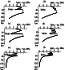Figure 2
The fractured waveguide structure is naturally interpreted in terms of the analysis of strength and fracture characteristics of rocks, which are determined from laboratory tests at appropriate pressures and temperatures and from various theoretical models. The very notion of the strength of crustal material needs to be additionally specified. The strength is usually understood in mechanics as a loading limit of an elastic-brittle or ductile material. However, the thus determined strength depends on the time and mode of loading, as well as on the scale of the study object. Therefore, it is not a an authentic characteristic of the material. The notion of creep strength related to a level of deviatoric stresses at a given strain rate is introduced under nonlinear viscous flow conditions. The strain behavior, the fracture mode and thereby the essence of the strength notion change with depth. For this reason, the so-called generalized strength, including all of the above aspects, is introduced. The generalized strength characterizes the compliance of material and its liability to fracture under real conditions at specific depths and at pertinent pressures. The physical meaning of this characteristic is somewhat indefinite, but it is very beneficial to the study of mechanical properties of the crust. Figure 2 plots the generalized strength versus depth variation [Ranalli and Murphy, 1987]. As seen from the figure, the generalized strength strongly varies with depth. The upper lithosphere (crust) is cold and strong. However, the generalized strength has local minimums at various depths, and the first minimum is usually observed in the middle crust. Figure 2a characterizes the lithosphere of Precambrian shields with a 40-km thick granite crust. The strength starts decreasing from depths of 20-25 km. Figure 2b plots the results calculated for a cold, normally thick continental crust stratified into granite (about 20 km thick) and basite layers. Weakened zones are observed at the boundary between them. Figure 2c presents the strength curve calculated for the cold continental lithosphere with a 60-km thick granite layer characteristic of Mesozoic-Cenozoic collision zones (Tien Shan and Tibetan plateau). The lower crust strength drop here is much larger than under the cold Precambrian shields. Figure 2d characterizes a situation similar to Figure 2c except that the crust is divided into granite and basite layers.

The picture is basically different in tectonically active regions, as is seen from Figures 2e, 2f. Figure 2e characterizes a 30-km thick continental crust under conditions of extension or shear (Basin and Range Province or San Andreas fault). The weakened brittle layer is at a depth of 10-15 km here. Given similar conditions and a crust stratified into granite and basite layers, two minimums appear at the bases of these layers (Figure 2f).

The weakened crustal zones fracture under strong shear deformations and develop into waveguides with fractured porous structure. This concludes the possible general description of the mechanism of waveguide formation. However, the generalized strength is too general and vague notion, and the actual fracture mechanism is more complex. Therefore, it is appropriate to consider other fracture schemes.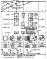Figure 3
Nikolaevskii  proposed a concept accounting for experimental results and observed crustal structure (Figure 3). The plots and diagrams shown in Figure 3 illustrate the classification the pre-fracture regime and the fracture itself as a function of depth. The upper part of the figure presents the ultimate stress, elastic limit and dilatancy onset versus pressure. The latter curve characterizes the ultimate shear at which the dilatancy expansion of the elastic medium begins as a result of cracking. The lower part of the figure presents experimental plots and diagrams of fracture, showing the fracture behavior as a function of pressure and temperature at various depths of the continental crust: the fracture mode alone varies with depth, whereas the fracture itself develops to depths as great as the lowermost crust. If the lateral compression is small, the cracks are parallel to the compressive stress. This accounts for the fact that fracture and major fault planes in the upper crust are subvertical. A system of inclined fractures develops at greater depths. This is consistent with experimental data indicating that inclined cracks arise in a sample at higher pressures. As the depth further increases and the Coulomb friction force becomes comparable with the ultimate strength, the stick-slip fracture due to the failure of material along the crack edges develops. Finally, the Coulomb friction force exceeds the ultimate strength, the fracture along major cracks becomes basically impossible, the entire medium becomes embrittled and deformations occur as a cataclastic flow. The zone of total fracture and cataclastic flow arises below the Conrad boundary; the state of material in this permeable and fractured zone can no longer change. The transition to the true plasticity (the medium is impermeable) occurs at the Moho.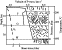Figure 4
Figure 4 shows the crustal structure as derived from seismic data (after [Bott, 1971]). The state transitions interfaces shown in Figure 3 correspond in Figure 4 to seismic boundaries F, C and M - Forsch, Conrad and Moho. The Forsch boundary is an interface marking the transition from inclined faults to the stick-slip (dilatancy) faulting.

This concept implies that crustal waveguides are identified with the stick-slip faulting zone between the Forsch and Conrad boundaries. The dilatancy effect associated with the pore volume enlargement takes place in this zone. Also, listric faults widen and flatten out here as is evident from various geophysical observations. The dilatancy expansion gives rise to the vacuum effect of sucking a fluid [Nikolaevskii, 1990]. Note that this scheme is rather formal and very approximate. It does not account for changes in the direction and value of tectonic stresses, as well as effects of fluids and dynamics of layers. In particular, the scheme does not account for the fact that the structure of layers is largely dependent on their preceding state, implying that the structure and the properties of the crust are described by evolutionary geomechanical equations including state diagrams. Therefore, the Nikolaevskii scheme should be regarded as a starting hypothesis providing very general ideas.

The displacement of overlying layers along a waveguide can be effected through a mechanism similar to lubrication. This is consistent with the concept of tectonic stratification [Tectonic Stratification..., 1980] underlying the idea of two-stage plate tectonics [Lobkovskii, 1988]. In accordance with these schemes, the crust consists of layers strongly differing in viscosity. The upper, most rigid part of the crust is divided into microplates moving relative to one another similar to macroplates in the classical scheme of plate tectonics. The problem of driving tectonic forces responsible for the motion of the system as a whole is also solved within the framework of the latter. These forces produce intraplate deformations and displacements of upper layers relative to lower ones. The energy of global motion is converted into the energy of regional movements of microplates which can be intricate and involve several levels, because not only waveguides but also the lowermost layers of the crust, involved in the cataclastic flow, can be plastic.

The permeability of rocks (particularly in fault zones) is known to be rather high, and their strength is low in such zones. Therefore, on a geological time scale, fluids should have been inevitably expelled out of waveguides, cracks and pores would have been closed and the waveguides themselves would have disappeared. Simple estimates show that the lifetime of waveguides in the upper crust is on the order of 10 2 -10 4 years. There are no reasons to believe that waveguides exist only at present. Apparently, they have existed since the origination time of the continental crust. Then, a driving mechanism should exist which periodically sucks fluids back into the waveguide, thereby enhancing the fracturing of the latter.

The assumption on viscous rheology of some crustal layers is supported by geological and geophysical evidence and is consistent with the concept of the rheological [Nikolaevskii and Sharov, 1985] and tectonic [Peive, 1981] stratification, as well as with the concept of two-stage plate tectonics [Lobkovskii, 1988].

### 1. Physical Properties of a Waveguide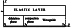Figure 5
The model proposed in [Karakin, 1990a; Karakin and Kambarova, 1997] is represented by a system of two thin layers (Figure 5) resting on a rigid impermeable substratum. The upper layer is elastic and has a low permeability. The lower layer (waveguide) is fractured and porous, is saturated with aqueous fluids and possesses certain specific properties of filtration and deformation.

The material of the lower layer can assume two states: dilatancy and compaction. The skeleton in the first state is elastic-brittle. The main factor controlling this regime is the Coulomb-Mohr law relating normal ( s ) and ultimate shear ( t ) stresses on a microlevel. When averaged and reduced to a macrolevel, this law is represented by certain relations connecting macroscopic quantities. Since the porous medium of waveguide is saturated, its stress state is defined by the Terzaghi principle, according to which the total stress tensor is divided into effective stresses sefij and pore pressure p: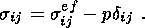(1.1)

The Terzaghi principle states that the state of material is defined by the effective stress tensor, or more specifically, by its isotropic part. Another independent parameter of state is the porosity f. The higher the porosity, the more prone the porous structure skeleton to fracture.

The vertical force applied to the base of the elastic layer is constant in the thin-layer approximation; it is independent of the stress state of the two-layer system (in particular, it is independent of the dilatancy expansion in the lower layer) and is equal to the elementary column weight of the elastic layer. The deviatoric stresses at the waveguide depth being small compared to the rock pressure, the latter is constant at the waveguide top and changes with depth in accordance with the geostatic law. Only the pore pressure p and the isotropic component of the effective stress tensor sefkk experience significant variations. These quantities are interrelated via relation (1.1) and therefore the pore pressure may be taken as an independent controlling parameter p.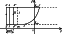Figure 6
I assume that the aforementioned properties of this model can be described in terms of a certain diagram of states. This diagram can be simplified proceeding from the following considerations. Formulating the problem in a vertical plane, I introduce a system of coordinates ( x, z ) with a vertical axis z directed upward. Using relation p = pa + rgz + const, the pore pressure can be divided into the geostatic component (equal to rgz + const ) and the piezometric component pa accounting for filtration. Here r is the density of the two-phase medium and g is the gravity acceleration. In view of the aforesaid, the values sefkk and pa coincide. In other words, the properties of the medium and the cyclic process at any point of the waveguide can be described in terms of the diagram of state in the plane ( pa, f ) shown in Figure 6, where two areas of states I and II are separated by the curve s(f) at which the skeleton structure experience a qualitative change.

An elastoplastic dilatancy regime takes place at pa < s. It is described by dilatancy equations which results from averaging the formation process of cracks obeying each the Coulomb-Mohr law. Due to shear loading under dilatancy mode conditions the pore space expands, the pore pressure decreases, and the waveguide sucks in fluids from both above and below. Faults play the role of drainage channels. This process is known in literature and has been repeatedly described as seismic (or tectonic) injection or as a "seismic pump.'' The crack-pore volume enlarges up to a maximum porosity value f max at which the porous structure skeleton fractures. The load is transferred at this moment from skeleton to fluid, and the pore pressure dramatically drops. At pa > sthe pore pressure is so high that the existence of a coherent skeleton is basically impossible: the skeleton disintegrates into separate grains that can move relative one another.

The waveguide material in this state is transformed into a porous-granular structure with a viscously deformable skeleton possessing both shear and bulk viscosities, and the deformation process is accompanied by fluid filtration. Such a medium is described by compaction (viscous consolidation) equations.

Drainage of the system in the compaction regime is very limited (because large cracks in outlet channels and faults are closed) and occurs through the low-permeability roof of the waveguide. Porosity in the compaction process decreases to its minimum value f = f min at which pores close. This is accompanied by resurrection of the skeleton from the nonviscous granular structure, the compaction regime is transformed into the elastic-plastic mode, and the cycle is further repeated.

I assume that the shear viscosity in compaction equations is constant and the bulk viscosity and hydraulic resistance (the inverse of permeability) are porosity dependent. Then, the compaction equations of motion are generally nonlinear. However, their solutions admit the principle of superposition of shear and volume deformations, i.e. these components of the solution can be treated independently of one another. Moreover, the volume deformations and filtration processes in the lower layer can be treated independently of the dynamics of the upper layer. The compaction phase duration is equal to the time required for pores to diminish under the action gravity from maximum to minimum sizes. This process determines the compaction phase time alone (and thereby the entire time interval of the wave motion) and does not affect the deformation mode of the two-layer system. The coupling of the upper and lower layers in the compaction phase affects shear deformations in the lower layer alone. Therefore, the elastic and viscous layers form a coherent system described by the Elsasser equation similar to the equation describing the two-layer asthenosphere-lithosphere system.

Thus, the pore space configuration changes at the maximum ( f max ) and minimum ( f min ) values of porosity. These states alternately change one another in a quasi-dynamic regime illustrated by the diagram in Figure 6. The cyclic structural variation is represented in this diagram by a closed contour. The single and double dashes indicate respectively the waveguide bottom and top in the dilatancy phase. The respective trajectory segments are vertical, because the pore pressure variation in the dilatancy phase are much weaker than in the compaction phase. The distance between these segments is DrgH1, where Dr the density contrast between the skeleton and fluid.

The entire system is set in motion by a horizontal force of tectonic origin applied to the upper elastic layer. This force supplies the energy to the entire process. To a first approximation, the upper layer is under the action of homogeneous horizontal normal stresses. The next approximation should account for tangential stresses and the gradient of normal stresses. The tangential stresses at the base of the elastic layer are in equilibrium with the normal forces in this layer and produce shear deformations in the lower layer. Thus, at any time moment the waveguide is under shear conditions.

The force of resistance of the lower layer to the upper one increases with the horizontal size of both layers and tends to infinity as the horizontal size infinitely increases. Accordingly, the normal tectonic force driving the system also tends to infinity. Nevertheless, the model equations admit a wave solution possessing periodical properties. Hence the necessity to separate periodic and aperiodic components of the solution and to correctly formulate the problem by specifying appropriate boundary and additional conditions.

Such a separation is based on the assumption that all nonstationary processes are periodic and the aperiodic component of the solution is stationary. The driving force of the process is represented by given stresses at infinity described by a static aperiodic component. These stresses and the work done by them are converted into a wave process. Consequently, these two components should be related through boundary conditions.

Note that the wave process complying with such an idealized formulation is unrealistic. In reality, a 3-D problem bounded in time and space should be solved. However, the possibility of a correct formulation of the problem and the existence of an idealized solution are basically important for understanding the physical nature of this process.

Upon separating a stationary, monotonically increasing component from the general solution of the two-layer system, a wave solution to the residual problem is sought for as follows. The dilatancy-mode porosity increases from its minimum to a maximum value. The compaction-mode porosity decreases from its maximum to the minimum value. The wave nature of the solution implies that the time t appears the wave argument x - ut, where u is the wave velocity found from the solution. Actually, the compaction equation alone includes the time derivative, so that the wave period is determined by the compaction phase duration. The wave solution can be considered existing if one succeeds in constructing a periodic solution for the wave argument. This process will be referred to as self-excited waves. Its driving force is provided by horizontal tectonic stresses. Due to the aforementioned specific properties of the waveguide material, the constant horizontal tectonic forces give rise to complex self-excited wave processes in the two-layer medium.

### 2. Analysis of the Upper Elastic Layer of the Two-Layer System

As mentioned above, the upper layer is described by equations of the elasticity theory. Vertically uniform horizontal forces directed along the positive direction of the x axis are applied at the lateral boundaries. The upper boundary is free and tangential stresses resulting from the interaction with the lower layer are applied at the lower boundary. I assume that the wave moves in the negative direction of the x axis. All parameters of a stationary wave depend solely on the argument y = x + vt. In the moving coordinate system, the definition region of length L is bounded by the vertical boundaries y1 < y < y3, L = y3 - y1 (Figure 5). The lower and upper layers of thicknesses H1 and H2 are defined by the respective intervals -H12. Since the hydrostatic component of the solution in the upper layer plays no role in the solving process, I subtract it from the initial equations. For definiteness, I address the plane strain state, implying that lateral displacements are absent but lateral stresses are present. Then,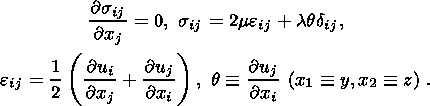(2.1)

Here, sij and eij are the stress and strain tensors, q is the isotropic part of the strain tensor, u is the displacement vector, and l and m are the Lame coefficients reduced to the plane case ( K = l + m is the reduced bulk modulus). Thus, the boundary problem for the upper layer reduces to the problem for a weightless elastic layer with certain forces applied to its boundary. Stresses vanish at the upper boundary of the layer, whereas stresses and displacements ui are continuous at its lower boundary. The displacements vanish at the lower boundary of the waveguide: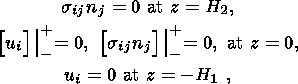(2.2)

where the symbol [sij]|+- means a jump in the quantity sij at a given boundary, nj is the normal unit length vector.

The wave process implies certain periodicity conditions that are authentically valid in the lower layer. In the upper layer all quantities include both periodic and aperiodic components. In other words, the horizontal stress sxx and displacements ux are represented as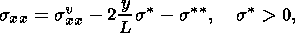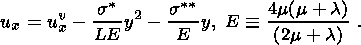Here, E is the plane analog of the Young modulus, and svxx and uvx are wave components of the horizontal stresses and displacements, which meet the periodicity condition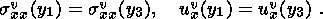(2.3)

The values sand scharacterize forces applied at infinity, which set the system in motion. More specifically, sdetermines the stress increase rate and sis related to the choice of the reference point. The minus sign at these values indicates that the forces are compressive. Strictly speaking, constant s, as well as stresses sxx, tends to infinity as the length of layers increases. However, they can be subtracted from these infinite stresses. In other words, the finite quantities sxx = sxx + sand ux = ux + 2sy/LE are introduced instead of sxx and ux; below the previous notation of these values is preserved (the tilde sign is omitted) because infinite stresses and displacements are meaningless and are no longer used. As a result, the boundary value problem considered in the interval y1 < y < y3 includes solely finite stresses and displacements which can be represented as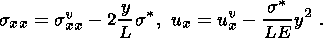(2.4)

However, one should keep in mind that in this case the values sxx and ux are no more than incremental stresses and displacements measured from a certain mean level (which is, generally speaking, infinite). By definition, we have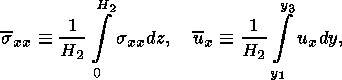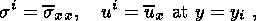where sxx and ux are laterally averaged horizontal stresses and displacements. The values si and ui are to be determined from the solution. By definition the wave components meet the conditions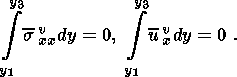(2.5)

Averaging (2.4) over the vertical profile and taking (2.5) into account yield additional relations for the average horizontal stresses and displacements: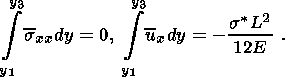(2.6)

Stretching the vertical coordinate z = ez in 2-D elastic equations (2.1), I obtain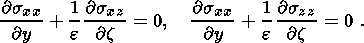Expanding the quantities in these equations into series sij = s(0)ij + es(1)ij +etc., simple argumentation leads to the relations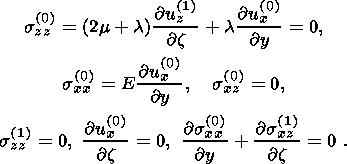(2.7)

Equations (2.7) imply that the horizontal displacement u(0)x and the normal stress s(0)xx are independent of the lateral coordinate z: u(0)x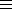u(x) and s(0)xxs(x). Integrating the last of equations (2.7) over the vertical profile and taking into account boundary conditions (2.2) yield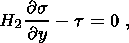(2.8)

where t is the tangential stress at the lower boundary.

Shearing forces are transmitted from the upper layer into the lower one, where they produce movements. Both possible states characterized by the diagram in Figure 6 can be realized as two phases: dilatancy (loosening the material) and compaction (or viscous consolidation, decreasing the porosity). Equations of motion and relevant boundary conditions are written out for each of these phases. The resulting solutions are adjusted to make them continuous at the boundary with the upper elastic layer and at the phase interface. In particular, the continuity condition imposed on the displacements and stresses at the phase interface in the upper layer is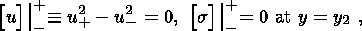(2.9)

where u2+ and u2- are the u values at the inner boundary when approached from the right and from the left, respectively.

Substituting the second expression from (2.7) into the first relation in (2.6) and taking (2.9) into account provide the periodicity condition for the displacement increment (2.3). Therefore, the first condition in (2.6) is not independent. Expanding the second relation in (2.6) into a series, I obtain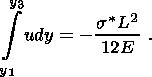(2.10)

Note that equation (2.10) contains the parameter srepresenting the increase rate of static stresses at infinity. It is actually a controlling parameter that describes the driving mechanism of the system.

### 3. Dilatancy Phase

As shown above, the lower layer is always under the shear conditions. Displacements in both dilatancy and compaction phases are measured from the stationary component which is not present in the constitutive equations of the phases. Both deviatoric and isotropic components of stresses and strains are assumed to be nonzero in both phases under shear loading conditions. In this case their interrelation is essential and includes the Coulomb condition and relationship between increments in shear gpepxz and volume strain qp:

dqp = bdgp,

where b is the dilatancy coefficient. The volume enlargement phenomenon associated with shear was experimentally established by Reynolds in 1885 and was called by him the dilatancy. The tensor dilatancy equations consistent with the conditions of Reynolds' experiment were formulated for the first time by Nikolaevskii [1967, 1971]. Afterward similar equations and their partial cases were examined by Rudnicki and Rice [Rice, 1980] and other authors (Rudnicki and Rice studied the problem of bifurcation in the solutions of these equations).

Following Rice , I introduce the plasticity moduli mp, lp and Kp similar to elastic moduli. To simplify the plastic strain case, I restrict myself to the shear strain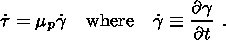(3.1)

The plasticity moduli depend on the drainage conditions. I assume that, in any dynamic processes, changes in the pore volume are small compared to its average. Then, the dilatancy-phase drainage conditions affect only the fluid regime in the upper layer and have no effect on the plastic deformation mode in the waveguide itself. This means that relation (3.1) includes an undrained shear modulus at any (loading or unloading) deformations. Another assumption is that the elastic strain component is neglected. Then the total strain coincides with the plastic component, and the dilatancy volume variation is only controlled by the porosity variation dq = df: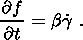(3.2)

The third assumption is the consequence of the first two and implies that the plasticity moduli mp, lp and Kp are small compared to their elastic analogs m, l and K. The physical meaning of these assumptions reduces to the statement that the fractured medium in the lower layer is in a subcritical state. This is the reason why the plasticity moduli and porosity variations are small, and plastic shear strains are very large.

The fourth assumption relates to the structure of the layers. I assume that the waveguide top is a low-permeability cap ensuring the conditions of weak drainage of the waveguide. It is due to this cap that, during a change in the state of the medium, the weight of the upper elastic layer is transferred at a critical point of the skeleton fracture from the skeleton to fluid, as is required for the regime of viscous consolidation in a medium with a fractured skeleton. If the cap mentioned above is absent, the waveguide is unloaded under conditions of elastic deformation. Such conditions are likely to exist in crystalline shields such as the Canadian and Fennoscandian shields. Ductile sedimentary rocks suitable for an impermeable cap are absent there. Therefore, the oscillatory process in such a waveguide does not include the compaction phase.

Substituting (3.1) into (3.2), I obtain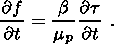(3.3)

Let the strain in the lower layer be measured from the state with a minimum porosity and a zero strain. The horizontal displacement at the top of the waveguide u is equal to the horizontal, vertically uniform displacement of the elastic upper layer. In each phase this horizontal displacement will be measured from an initial point at which a given phase starts developing. The initial point in the dilatancy phase is y1 and the initial displacement is u1. The respective values in the compaction phase are y2 and u2. These values are evidently connected through the kinematic relation u - u1 = H1g, which allows easy integration of equations (3.2) and (3.3):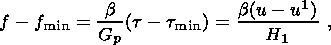(3.4)

i.e. as shear strain increases in the waveguide zone, porosity f and shear stress t increase from their minimum values f min and t min to critical levels f max and t max triggering the fracture of the skeleton and the transition into the viscous phase in accordance with the criterion illustrated in the diagram of Figure 6 and based on the Terzaghi principle. If the critical porosities f min and f max are known from the diagram in Figure 6, t min and t max are values found in the process of solution. Equation (3.4) leads to the relation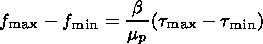(3.5)

relating t max and t min.

The aforesaid implies the validity of the following relations at the boundaries between dilatancy and compaction zones: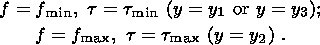(3.6)

The porosity f = f max is the initial value of this parameter in the compaction phase. The shear in the lower layer is connected with the displacement of its upper boundary through the relation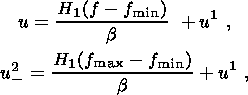(3.7)

where u2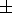denotes values assumed by u2 when the phase interface is approached from the right and from the left, respectively.

Combining the second relation in (2.7), (2.8) and (3.4) yields the equation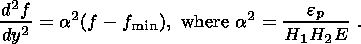(3.8)

Solving (3.8) under condition (3.6) gives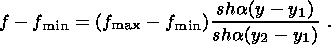(3.9)

Note that a(y2 - y1)1. Since E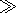mp, the extent of the dilatancy phase y2-y1 is much greater than the layer thicknesses H1 and H2. Using (3.9), (3.7) and the second relation in (2.7), expressions for displacement and stresses can be derived: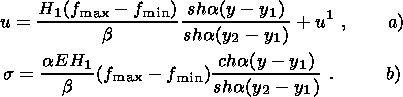(3.10)

These expressions give the boundary values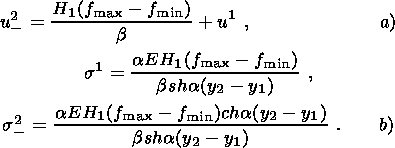(3.11)

Relations (3.11) connect the dilatancy phase length with amplitudes of stresses at the initial wave point.

### 4. Compaction Phase

According to [Karakin, 1990b, 1999], the compaction equations admit the superposition principle, so that shear and volume strain can be treated independently of one another. First I address shear motions in the compaction zone that can be matched to shear motions in the dilatancy zone. The calculations performed for the elastic layer are all valid for the compaction zone. However, they are different in the lower layer experiencing viscous deformations. In particular, (3.3) is replaced by a similar equation for viscous deformations:

t = h1 g.

Combining this equation with (2.8) and the second equation in (2.7) yields the parabolic equation for a two-layer system derived by Elsasser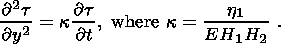The substitution of the wave argument in these two relations yields the equations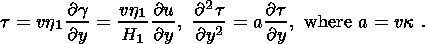(4.1)

The latter of these equations is readily solved with boundary condition (3.6):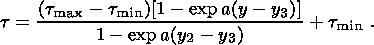(4.2)

As expected, the tangential stress in the compaction zone changes from its maximum to minimum values. Using (2.8), (4.1) and (4.2), I obtain expressions for the normal stress and its boundary value at the inner boundary: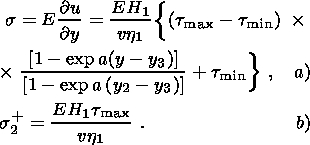(4.3)

Integrating (4.3a) and taking into account the periodicity condition for displacements (2.12) yield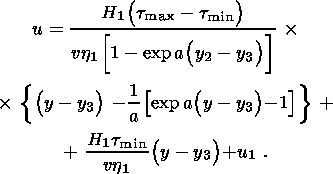(4.4)

Displacement continuity condition (2.9) at the inner boundary, with (4.4) and (3.7) taken into account, leads to the equation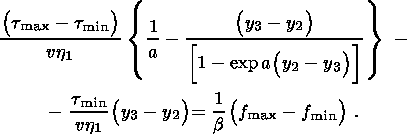(4.5)

Taking account of (3.10) and (4.3b), a similar relation follows from the normal stress continuity condition (2.9):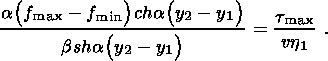(4.6)

Hence v > 0, i.e. the wave (as expected) travels in the direction opposite to the direction of the tectonic force applied. Eliminating the indefinite values t min and t max in (3.5), (4.5) and (4.6), I obtain the relation connecting the lengths of the compaction and dilatancy phases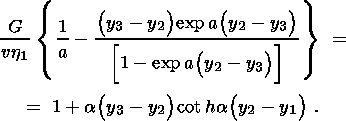(4.7)

The periodicity condition for the normal stresses gives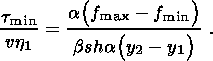(4.8)

The substitution of (4.4) and (3.10a) into (2.10) yields the expression determining u1: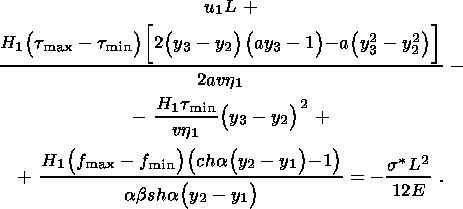(4.9)

Let Tc be the time of compaction obtained below from the solution of the boundary value problem of compaction. It is connected with the wave velocity through the relation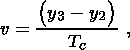(4.10)

which allows one to determine the compaction phase length ( y3 - y2). The solution of equations (3.5), (4.5), (4.6), (4.8), (4.9) and (4.10) determines the six sought-for quantities u1, t min, t max, (y2 - y1), (y3 - y2) and v, expressed through Tc. This reduces the final solution of the problem to the determination of the compaction time Tc, which is found from the solution of the 1-D compaction problem.

In this connection, I consider a 1-D compaction problem in the finite region -H1 < z1 < z < 0 with a moving boundary of the trailing edge type described by the function z1 = -H(x, t), where H(x, t) is the thickness of the compaction layer. Following [Karakin, 1999], equations of 1-D low-porosity compaction have the form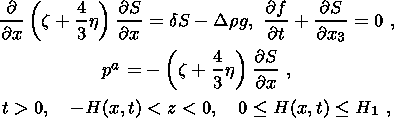(4.11)

where S is the filtration flow, f is porosity, d is the hydraulic resistance coefficient, and z and h are coefficients of bulk and shear viscosity. The layer thickness is variable and depends on the horizontal coordinate x as a parameter. The lower boundary of the viscous consolidation zone G1 can be either movable or fixed, depending on the viscosity value. Various motion regimes are possible in the nonstationary case. In particular, it is fixed at f > f min and then the following condition is valid at this boundary: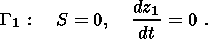(4.12)

When the porosity at this boundary attains the value f min, the boundary starts to move upward until it encounters the upper boundary G2. The movement of the stationary wave is only consistent with the trailing edge condition at the lower boundary: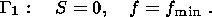(4.13)

The upper boundary of the waveguide G2 is fixed. It is overlain by a porous layer with an elastic skeleton. The boundary value problem of elastic consolidation (the diffusion equation) should be solved, and the resulting solution should be joined to the solution in the waveguide. However, for the purpose of qualitative analysis, the joining condition can be replaced by a simpler constraint: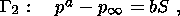(4.14)

where pis the pore pressure in the upper layer far from its boundary ("at infinity''). This condition has the following meaning. The characteristic time of the elastic consolidation (estimated from the diffusion equation) is much shorter than the viscous consolidation time. Consequently, this condition does not contain the time derivative and is similar to the heat transfer relation for the heat conduction equation. The constant b is expressed through geometrical and filtration characteristics of the region with elastic skeleton overlying the waveguide. The filtration flow direction changes its sign at p < pand therefore vanishes at this point. Then, condition (4.14) provides qualitatively correct, albeit gross, constraints on interaction of the two media at the boundary G2. The system of boundary conditions also includes the initial condition for porosity. As mentioned above, this condition is determined by the porosity at the dilatancy-to-compaction transition moment and has therefore the form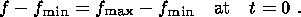(4.15)

The initial time moment coincides with the onset time of the compaction phase for each oscillation cycle. Because the equations of shear and volume strain split into two groups, the time enters each of these groups independently. The boundary value problem (4.11)-(4.15) was numerically solved, and the procedure and results of the solution are presented in [Karakin and Levitan, 1993]. The time during which the length of the compaction region decreases to zero is shown to be finite and is the compaction period Tc. This concludes the solution of the problem.

Boundary condition (4.14) determines the across-waveguide difference of pressure, which is greater than or on the order of DfK, where Df is the total change of the porosity over the entire cycle of oscillation in the waveguide and K is the elastic bulk modulus of the upper layer. Since the dilatancy bulk modulus Kp is much smaller than K, the pore pressure varies in the dilatancy phase much weaker than in the compaction phase. For this reason, the dilatancy phase trajectories of the diagram in Figure 6 and phase interfaces are vertical.

### 5. Conclusion: Vertical Migration of Fluids in the Upper Crust

Thus, a self excited wave regime of motion can arise in a two- layer system subjected to the action of a force. This regime is related to periodic processes of fluid expulsion from and suction into the waveguide layer. The compaction phase is much longer than the phase of dilatancy expansion. The interaction between these phases maintains the state of dynamic equilibrium in the waveguide.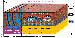Figure 7
The functioning of the self-excited wave mechanism of the fluid motion in the waveguide and overlying layers is schematically illustrated in Figure 7. In the dilatancy phase, downgoing fluid flows entraining hydrocarbons In the dilatancy phase, downgoing fluid flows entraining hydrocarbons enter the waveguide through listric faults extending into it. The solubility of hydrocarbons in water being very low (less than 1%), they are transported in the emulsion form in the upper crust where the porosity in listric faults is rather high. Thermodynamic conditions at waveguide depths are such that their solubility (particularly, of their gaseous phase) increases. This is beneficial to the accumulation and concentration of hydrocarbons in the waveguide zone. Although the relative amount of hydrocarbons transported over the individual wave cycle is small, the amount of hydrocarbons entrapped in the waveguide on the geological time scales and over large areas is very large.

As is known, the theory of organic origin of oil encounters basic difficulties. In terms of this theory, hydrocarbons derive under suitable thermodynamic conditions from organic matter uniformly dispersed throughout the sedimentary cover and concentrate to form accumulations relatively small in volume. This is a paradoxical fact. At first glance, it contradicts thermodynamics, according to which all substances in the dispersed phase tend to spread rather than concentrate. The problem of resolving this paradox arises. This problem, albeit in a less explicit form, also exists in the theory on inorganic origin of oil. Similar to the first case, ascending hydrocarbons of the endogenic origin should uniformly disperse throughout the crust.

Another paradox is that advocates of both organic and inorganic origin of hydrocarbons present equally convincing arguments in favor of their hypotheses. Thus, both hypotheses seem equally acceptable, and no unbiased criterion for the choice of them exists.

These contradictions and paradoxes can be resolved if one supposes that fluids in the crust are involved in oscillatory movements and repeat many times their trajectories, both vertically and horizontally. In this case the origin of hydrocarbons is no longer the problem of basic importance and is unrelated to the mechanism of their concentration.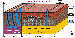Figure 8
Fluids in the compaction phase are expelled upward from a waveguide. Their motion can be obstructed by impermeable anticlinal structures (traps), thereby developing abnormal formation pressure. Traps are usually formed by anticlinal folds of impermeable rocks. If ascending fluid flows strike concentrated hydrocarbons, conditions for their accumulation arise. If the crest of an anticlinal structure is cut by faults (Figure 8), fluids rush upward through the fault zones and are ejected on the Earth surface, forming gas outbursts and mud volcanoes. According to the hypothesis proposed in this work, real oil and gas fields and mud volcanoes have a common origin. The only distinction of mud volcanoes is that their traps are fractured. This accounts for the fact that mud volcanoes gravitate toward faults and shelf flexure zones, where impermeable beds are most prone to fracturing.

The concept proposed explains many facts, in particular, the position of mud volcanoes in rapidly subsiding basins at anticlinal crests cut by faults. Fluid flows breaking through anticlinal trap roofs can entrain hydrocarbons and mud-volcanic breccia. They form mud volcano vents distinguished by weak seismic contrast.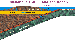Figure 9
Using this concept, the motion of a two-phase gas-liquid mix was numerically modeled for the upper crust of the Varandei-Adzvinskaya oil-and-gas province [Dmitrievski et al., 2000]. In particular, a numerical model was developed for the formation process of gas fields in this region. A distinctive feature of the geological structure and geodynamic development of this region is the fact that it a former subduction zone that developed as a result of the closure of an ancient ocean. Huge masses of sedimentary rocks were pulled into interiors in the plate collision process at passive margins. These masses contained large amounts of organic matter and are presently oil source rocks, which have a large potential for the formation of hydrocarbon deposits. However, its realization requires a driving mechanism. Such a mechanism is represented by the self-excited wave regime of motions in the crustal waveguide. Figure 9 schematically illustrates how the material of passive margins is pulled down in a subduction zone at a passive margin. Crustal waveguides that cross subducting lithospheric plates in the horizontal direction form even during the subduction process.

Hydrocarbon deposits of the Sakhalin shelf type can also develop in terms of this model. As in the case of continental deposits, traps cut by faults give rise to mud volcanoes rather than hydrocarbon deposits (Figure 9). However, as distinct from continental volcanoes, liquefied serpentinite rather than a sand-clay mix is erupted in deep-sea trenches of subduction zones.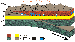Figure 10
Some time after the subduction stops, an ex-collisional structure similar to the Varandei-Adzvinskaya oil-and-gas province develops in the former subduction zone (Figure 10). A distinctive feature of subduction and particularly excursion-collision zones is their typical across-strike differentiation illustrated in Figure 10. Gas deposits are located in the frontal part of the zone, corresponding to the sinking slab. Heavy oils and bitumen occur in the back zone, and oils of intermediate composition accumulate in the middle part of the zone. This composition-type differentiation hydrocarbon deposits is completely consistent with the driving mechanism of fluid motion in the crustal waveguide. In the frontal part, fluids enter the waveguide from below at high temperatures and pressures. This is beneficial to the segregation of large amounts of gas fraction from oil source rocks. In the back part, oil source rocks overlying the waveguide are flushed at low temperatures and pressures, which naturally leads to the formation of heavy oils and bitumen.

### References

Barcilon, V., and O. Lovera, Solitary waves in magma dynamics, J. Fluid Mech., 204, 121-133, 1989.

Bott, M. N. P., The Interior of the Earth, Edward Arnold, London, 1971.

Dmitrievski, A. N., I. E. Balanyuk, Yu. A. Poveshchenko, A. V.  Karakin, and M. I. Lodzhevskaya, Mechanism for hydrocarbon deposit emergence, Gas Industry of Russia, Digest, p. 5-9, 2000.

Elsasser, W. H., Two-layer model of upper mantle circulation, J. Geophys. Res., 76, 1101-1112, 1971.

Feldman, I. S., On the nature of conductive layers in the Earth�s crust and upper mantle, Geoelectr. Geotherm. Stud. KAPG Geophys. Monogr. Bp., 721-745, 1976.

Fyfe, W. S., N. J. Price, and A. B. Thomson, Fluids in the Earth's Crust, Elsevier, Amsterdam, 1978.

Gordin, V. A., and A. V. Karakin, Solitary wave solutions in problems of viscous consolidation, Matem. Model., 2, (2), 98-116 (in Russian).

Grigoryev, S. M., The Role of Water in the Earth Crust Formation (Drainage Envelope of the Crust), Nedra, Moscow, 1971 (in Russian).

Gutenberg, B., Physics of the Earth's Interiors, Academic Press, New York, 1959.

Karakin, A. V., A model of the fluid motion in the crust over geological time intervals, Mate. Model., 2, (3), 31-42, 1990a (in Russian).

Karakin, A. V., Crustal fluid dynamics models with an inelastic skeleton, Izv. Akad. Nauk SSSR, Fiz. Zemli, (2), 3-15, 1990b (in Russian).

Karakin, A. V., General theory of compaction with a low porosity, Fiz. Zemli, (12), 13-26, 1999 (in Russian).

Karakin, A. V., and L. I. Lobkovskii, Mechanics of a porous two-phase asthenosphere, Izv. Akad. Nauk SSSR, Mekh. Zhidk. Gazov, (6), 53-63, 1979 (in Russian).

Karakin, A. V., and S. Yu. Levitan, Modeling of fluid dynamics processes in rocks with a viscous skeleton, in Mathematical Modeling of Geological Processes, p. 17-32, VNIIGeosystem, Moscow, 1993 (in Russian).

Karakin, A. V., and G. N. Kambarova, A dynamic model of crustal waveguides, Geoinformatika, (4), 10-17, 1997 (in Russian).

Krasnopevtseva, G. V., Geological and geophysical structural features of low-velocity zones in the crust. An Overview: Regional, Exploration and Well Logging Geophysics, VIEMS, Moscow, 1978 (in Russian).

Lobkovskii, L. I., Geodynamics of Spreading and Subduction Zones and Two-Stage Tectonics of Plates, Nauka, Moscow, 1988 (in Russian).

Magnitsky, V. A., Low-Velocity Zone in the Upper Mantle, Nauka, Moscow, 1968 (in Russian).

McKenzie, D., The generation and compaction of partially molten rock, J. Petrol., 25, 713-765, 1984.

Nikolaevskii, V. N., Relationship between volume and shear plastic deformations and shock waves in soft soils, Dokl. Akad. Nauk SSSR, 177, (3), 542-545, 1967 (in Russian).

Nikolaevskii, V. N., Constitutive equations of plastic deformation in Loose Ground, Prikl. Matem. Mekh., 36, 1017-1029, 1971 (in Russian).

Nikolaevskii, V. N., Mechanics of Porous and Fractured Media, World Scientific, Singapore, 1990.

Nikolaevskii, V. N., and V. I. Sharov, Faults and rheological layering of the crust, Izv. Akad. Nauk SSSR, Fiz. Zemli, (1), 16-27, 1985 (in Russian).

Overdeep Kola Drillhole, Nedra, Moscow, 1984 (in Russian).

Peive, A. V., Mobilism and tectonic stratification of the lithosphere, Priroda, (2), 2-9, 1981 (in Russian).

Ranalli, G., and D. C. Murphy, Rheological stratification of the lithosphere, Tectonophysics, 132, 281-295, 1987.

Rice, J. R., Mechanics of Earthquake Rupture, Amsterdam, 1980.

Seismic Models of the Lithosphere under Main Geological Structures of the USSR, Nauka, Moscow, 1980 (in Russian).

Smith, F. G., Physical Geochemistry, Nedra, Moscow, 1968 (in Russian).

Tectonic Stratification of the Lithosphere, Nauka, Moscow, 1980 (in Russian).

Turcotte, D. L., and G. Schubert, Geodynamics, Applications of Continuum Physics to Geological Problems, Wiley, 1982.

Vanyan, L. L., Electrical conductivity of the crust in relation to its fluid regime, in Crustal Anomalies of Electrical Conductivity, p. 27-34, Nauka, Leningrad, 1984 (in Russian).

Vanyan, L. L., and P. P. Shilovskii, Deep Electrical Conductivity of Oceans and Continents, Nauka, Moscow, 1983 (in Russian).

Load files for printing and local use. This document was generated by TeXWeb (Win32, v.1.3) on October 31, 2000.